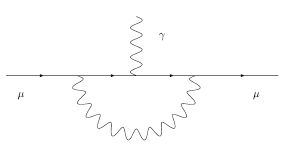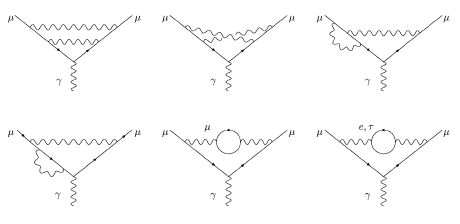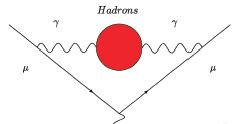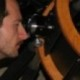## The muon anomaly and the Higgs mass – part I June 10, 2008

Posted by dorigo in physics, science.
Tags: , ,

Note: despite the technical nature of the matter, I have made an effort to keep this post to a level simple enough that non-scientists should be able to handle. Feedback is welcome!

Nowadays when you are presented with a statement about the inner consistency of the Standard Model of particle physics (SM), and on the range of mass values that a neutral Higgs boson may possess in order to
fit the observed value of several fundamental quantities -all related in a non-trivial way among each other- you are entitled to raise both eyebrows.

Indeed, theorists today speak of the SM as a still-dead entity, because they know it cannot be the ultimate theory. They say it only describes things so well because we have not tested it at energies and forces large
enough. They are quick to point out, if requested (or even without any prodding) that the SM is just “an effective theory”, meaning the opposite (with theorists this happens, at times -it is a lingo barrier rather than backward thinking). They may add that the SM fails to explain the smallness of the mass of the Higgs boson (which has in earnest no apparent reason to be as light as the model wants it), and it does not grant a high-energy unification of fundamental forces in a way which is pleasing to their eye, with the three coupling constants meeting at a single, very large energy scale.

The Standard Model does have those shortcomings. But it has survived more than thirty years of painstaking scrutiny. So your eyebrows have to come down once you realize that, despite all caveats, the predictive power of the combination of existing theory and excellent determination of its free parameters is astonishing. It ain’t no string theory!

There are dozens, but one might say hundreds, of experimental predictions that can be worked out, only to find the SM in exceedingly good health. It is thus not surprising that a handful of these predictions has shown some nagging disagreement with the data in the past. Among them, one might quote a few that are still unresolved today (each of them representing a deviation of measurement from theory by roughly two to three standard deviations): if you accept a list without explanation, I may quote the inconsistency of the measured value of the W boson mass with the observed ratio between neutrino charged-current and neutral-current interactions measured by the NuTeV experiment; the Z boson asymmetry measured by LEP, which shows a difference when measured in leptonic versus hadronic decays; the branching ratio of$Z \to b \bar b$ decays; and the anomalous magnetic moment of the muon.

The anomalous magnetic moment of leptons

A magnetic moment is a property of charged particles with a non-zero value of spin. Although quantum mechanics prevents us from drawing a perfect analogy, a spinning charged sphere develops a magnetic field, and so do charged elementary particles. For them the magnetic moment is easily computed as the product of charge by spin, divided by mass.

The so-called gyromagnetic ratio$g$ is then a pure number defined as the magnetic moment$\mu$ computed in units of its charge divided by twice its mass: for electrons$g_e =\mu / (e/2m_e)$ (where$e$ is the electron charge). The magnitude of$g_e$ describes the magnetic properties of the electron. All charged leptons have gyromagnetic ratios very close to 2, but not quite equal to it. They are exactly 2 in Dirac’s theory of charged fermions, but quantum corrections cause a shift. The deviation of g from 2 -its residual from it- is called anomaly, and it is universally recognized as a very important number,$a_l = (g_l-2)/2$. It is a crucial quantity in electrodynamics, and in particle physics in general, because it is a very small number which can be measured directly, and small non-zero numbers can usually be measured with high accuracy.

Indeed, the electron anomaly$a_e = (g_e-2)/2$ is measured with exquisite precision: it is found that$a_e=1159652180.73\pm28 \times 10^{-12}$, or to within 0.24 parts per billion! It is by its measurement that we know the value of the fine structure constant,$\alpha$ -the fundamental quantity of quantum electrodynamics. Theoretical predictions for$g_e$ can be computed to fractions of a billionth too, so a direct comparison of the two provides a spectacular test of our understanding of the underlying physics.

For muons,$a_\mu$ has been measured with accuracy better than five parts in ten millions, and here theory and measurement differ by 3.4 standard deviations. A paper by Massimo Passera and collaborators, which I will describe in detail tomorrow, discusses the discrepancy and critically analyzes it in terms of the consistency of electroweak fits and low-energy measurements used as input. What I want to do today is to give some preliminary information about the problem, so that I have a chance of explaining to outsiders the details tomorrow.

Calculating the muon anomalySo, what is it exactly that goes in the calculation of the muon anomaly? Well, it boils down to adding together the contributions of different processes which modify the Dirac picture of a muon (whose momentum is labeled “p” and then “p'”) emitting a photon, as in the graph on the right. At “leading order” -that is, when ignoring everything else but the bare-bone electromagnetic process of photon emission- the gyromagnetic ratio is 2 and the anomaly is zero. However, in subatomic physics every process that is not forbidden will happen, with a certain probability which is the square of the “amplitude” of the corresponding particle diagram. Looking beyond the bare-bone process, at “higher order” one needs to consider a huge number of other processes, such as the emission of a second photon by the muon line, with the former subsequently reabsorbed by the muon after the emission of the main outgoing photon, as in the top left graph of the figure below.As the number of allowed vertices increases, there may be two photon emissions, and fancier things may start happening, as shown below:Here we have to count at the same order (because they have the same number of vertices -points where three lines meet) diagrams where a single photon is emitted and reabsorbed, but the photon spends some time in the form of a virtual loop of charged leptons, as in the two graphs shown in the lower right above. At still higher orders the diagrams to consider are many more, but they respect the general structure with lines and blobs like those shown in the figures above.

Similarly, we can imagine that the incoming muon emits and reabsorbs a virtual Z boson; or the muon may emit a W boson and temporarily turn into a muon neutrino, as in the diagram in the center in the figure below. It is only by computing each and every possible virtual diagram, with all known particle interactions, that the total quantity we have to compute -the muon anomaly- comes out right.Of course, the number of diagrams diverges as the number of “vertices” (points where particle lines intersect) increases. But physicists are good at performing approximations: by organizing the “higher order” corrections in series of the number of vertices, they can prove that each additional term in the series is a small correction to the former. So they just continue calculating more and more complex diagrams until they have to give up (since the number of diagrams to be computed grows factorially with the number of vertices), and their final result will be good to within the estimated contribution of the first neglected term in the series.From the “nuts and bolts” description I gave above you have by now realized that in the “master” diagram we considered -photon emission from a charged muon line-, strong and electroweak physics enter by necessity at higher order in the perturbation series. Thanks to their electrical charge, even quarks may be produced by a photon fluctuation, and quarks are subject to strong interactions: what they may do, while they are alive in the red blob shown in the graph on the right, needs to concern us. The strength of those interactions will affect the final result for the muon anomaly despite the virtual nature of the quarks! The same goes with W and Z bosons which a muon line can emit (W bosons also connect to photon lines, thanks to their electric charge).

Thus,in the calculation of higher orders of the muon anomaly, there enter not only electrodynamics (which we claim to know inside-out), but also weak and strong interactions: the former are those mediated by the exchange of weak vector bosons (W and Z), the latter are instead those governing the dynamics of quarks -the constituents of nuclear matter- and gluons, the carrier of strong force.

The weakness of an interaction means that as we go to higher orders in the perturbation series diagrams with more vertices become very improbable, and the corrections they cause become small very quickly: the
series converges, and we can calculate it [Post-scriptum: The series does not actually converge – this is a mistake I prefer to not correct, see the comments thread below- but the calculation does work for quantum electrodynamics!]. But for quantum chromodynamics -QCD, the theory of strong interactions- this unfortunately does not happen! Alas, the basic QCD processes we need to compute are
“non perturbative”: higher-order contributions are large and cannot be neglected, no matter how you reorganize your perturbation series.

QCD is a wonderful theory, and high-energy processes can be computed with it with great precision, because at high energy the strong coupling constant (a number by which the probability of any QCD process has to be multiplied once for every particle vertex in the diagram describing the process) is small; but at low energy$\alpha_s$ is large, and perturbation series diverge.

Because of that nasty property of QCD, a calculation of the muon anomaly needs to rely on approximations, modeling, and the knowledge of low-energy QCD. Some processes that help derive the quantities which are used in these approximations are those we measure in low-energy electron-positron scattering experiments: the cross section of these reactions determine how strong an impact some QCD virtual diagrams have in the muon g-2 calculation.

Ok, I think I have dumped above the preliminary information one needs to have in order to read the next, I hope enlightening, post, which will discuss the recent analysis by Massimo Passera and collaborators. In their paper they explain how the upper theoretical bound on the mass of the Higgs boson depends on the amount of uncertainty in low-energy hadronic cross sections one is willing to allow. Those who can’t wait for the post, and can read a hep-ph paper without assistance, are encouraged to get it here.

UPDATE: This link will bring you to the second part of this post.1. Kea - June 11, 2008

I see their abstract (please fix your link) offers a solution and then the statement leading however to a decrease on the electroweak upper bound on M_H, the SM Higgs boson mass. Ah, the fairy field is really beginning to bite! Great post. You should win a science blogging award.2. Simon - June 11, 2008

Hi Tommaso, another interesting post! I always enjoy reading the point of view of someone who actually connects QFT with the ‘real world’. I just have one small question/comment:
You say that “…they can prove that each additional term in the series is a small correction to the former.” but I thought that Dyson, in 1951, proved/argued that this can not be the case. In a small 2 page article he argued the best that the perturbation series can be is asymptotic. Am I misunderstanding something?
http://www.slac.stanford.edu/spires/find/hep/www?j=PHRVA,85,631
btw It’s amusing to note that he published this argument less than a year after he (almost) proved renormalisability of QED!3. dorigo - June 11, 2008

Hmm the link works for me … What do you mean ?
Thanks for the encouragement btw. Today I will write about the paper.

Cheers,
T.4. dorigo - June 11, 2008

Hi Simon,

making things trivial means distorting them a bit. I am just trying to make the point that the series converges: I do not even want to mess with renormalizability in the post… Too much stuff already there.
In any case, you might be surprised to know that I had never seen Dyson’s argument. I am not a QFT expert: perhaps that is why I feel so comfortable in explaining it in simple terms 😉

Anyway, thank you for the note.

Cheers,
T.5. Georg - June 11, 2008

Sadly enough, the series does not converge. Key words are asymptotic series, and renormalons.6. dorigo - June 11, 2008

Hi Georg,

ok – I now read (better say browsed) the paper by Dyson, and I admit that the presentation above has an incorrect statement, which Simon pointed out well. I do not want to correct the text, however, because this is not a blog striving for accuracy, but rather attempting at presenting things in a simple way. Any reader interested in the matter will no doubt check this comments thread and get informed about my mistakes, in any case…

Cheers,
T.

PS I added a post-scriptum in the post, pointing at this thread…7. big vlad - June 11, 2008

great post!

Regarding the fact that the perturbation series diverges… this is something that I’ve often wondered about. What exactly does it mean to say that it does not converge, but instead is asymptotic? If higher order terms become large then it’s game over for perturbation theory, so presumably this isn’t the case (for well defines observables anyway).8. Georg - June 11, 2008

roughly speaking, an asymptotic series is a series which diverges for all values of the expansion parameter, but which gives an arbitrarily good approximation to some function for a sufficiently small value of the expansion parameter when a sufficiently large (finite) number of terms is retained. In fact, as long as the terms keep getting smaller, the asymptotic series keeps giving a better approximation to the underlying function. When a term first gets bigger, the error made in truncating at the smallest term is no larger than the first discarded term (given various highly technical conditions, which I can’t really recall).

A simple example is phi^4 theory in zero dimensions, also known under the less fancy name of the integral Z(\lambda) = \int_\{-\infty}^\infty e^{-1/2 x^2 -\lambda/4! x^4} dx. If you expand the integrand in \lambda and integrate term by term, you get an asymptotic series for Z. You can also evaluate the integral analytically (it gives some kind of Bessel function of fractional order), which allows you to compare the analytic form with the “perturbative” series and get an idea of how asymptotic series behave.

In fact, the study of asymptotic series is a mathematical discipline of its own, and there are various rigorous theorems about it. Of course, in nature \alpha\approx 1/137 is really a constant, not an arbitrary parameter, so these theorem don’t apply in a rigorous mathematical sense, but from a physics point of view, the things we know about asymptotic series are good enough to work with them without being too worried.9. dorigo - June 11, 2008

thank you for this discussion. The technological details of these calculations have always escaped me, so I am quite happy to read some simple explanation by Georg… This blog serves the purpose of boosting my own instruction sometimes.

Cheers,
T.10. island - June 11, 2008

Ah, the fairy field is really beginning to bite!

But chasing them continues unabated.

11. Massimo Passera: the muon anomaly and the Higgs mass - part II « A Quantum Diaries Survivor - June 11, 2008

[…] In the first part of this post I gave some sort of background information on the physics of the muon anomaly, at a level which I hoped would keep non-physicist readers alive through the end. That first part now helps me to write a summary of Massimo’s talk in a way which is hopefully both understandable and lightweight. Let us see what I manage below, where I make use of some concepts I explained yesterday. […]12. phenomenologist - June 12, 2008

Georg, thanks for your detailed reply. I’m still a bit confused about the definition of an asymptotic series. If it “diverges for all values of the expansion parameter”, how can it also give “an arbitrarily good approximation” to something?

apologies if I’m being dim here. Your suggestion of learning about this through phi^4 theory in 0 dims looks interesting, if I get time I’ll investigate…13. Georg - June 13, 2008

Hi phenomenologist,

a convergent series will eventually give an arbitrarily good approximation anywhere within its circle of convergence, and that approximation will only become better as you include more and more terms. An asymptotic series on the other hand will only give an approximation that is good up to an error of $\epsilon$ (where $\epsilon>0$ can be arbitrarily small) within some small interval $[0;\delta(\epsilon)]$, where $\delta(\epsilon)$ goes to zero as $\epsilon$ goes to zero, and only if you retain $N(\epsilon)$ terms. So while you can get arbitrarily close to the function, the range of arguments that you can do so gets smaller the more precise you want to be. Also, including more and more terms makes the approximation become worse even in that small interval if you go beyond beyond $N(\epsilon)$ terms (it diverges entually). Those series are weird, to be sure! For a more formal treatment than I am able to give, I am afraid have to refer you to the mathematical literature.

Sorry comments are closed for this entry